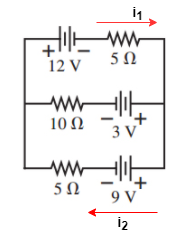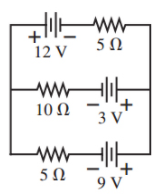# Problem: Part A. What is the current through the 10 Ω resistor in the figure (Figure 1)? Express your answer to two significant figures and include the appropriate units. Part B. Is the current from left to right or right to left? A. left to right B. right to left

###### FREE Expert Solution

Consider the direction of the currents as follows:Kirchhoff's voltage law:

$\overline{){\mathbf{\Sigma }}{\mathbf{V}}{\mathbf{=}}{\mathbf{0}}}$

97% (20 ratings)###### Problem DetailsPart A. What is the current through the 10 Ω resistor in the figure (Figure 1)? Express your answer to two significant figures and include the appropriate units.

Part B. Is the current from left to right or right to left?

A. left to right

B. right to left